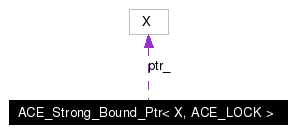# ACE_Strong_Bound_Ptr< X, ACE_LOCK > Class Template Reference

This class implements support for a reference counted pointer. More...

`#include <Bound_Ptr.h>`

Collaboration diagram for ACE_Strong_Bound_Ptr< X, ACE_LOCK >:[legend]
List of all members.

## Public Member Functions

ACE_Strong_Bound_Ptr (X *p=0)
ACE_Strong_Bound_Ptr (auto_ptr< X > p)
ACE_Strong_Bound_Ptr (const ACE_Strong_Bound_Ptr< X, ACE_LOCK > &r)
Copy constructor binds <this> and <r> to the same object.
ACE_Strong_Bound_Ptr (const ACE_Weak_Bound_Ptr< X, ACE_LOCK > &r)
Constructor binds <this> and <r> to the same object.
~ACE_Strong_Bound_Ptr (void)
Destructor.
void operator= (const ACE_Strong_Bound_Ptr< X, ACE_LOCK > &r)
Assignment operator that binds <this> and <r> to the same object.
void operator= (const ACE_Weak_Bound_Ptr< X, ACE_LOCK > &r)
Assignment operator that binds <this> and <r> to the same object.
bool operator== (const ACE_Strong_Bound_Ptr< X, ACE_LOCK > &r) const
bool operator== (const ACE_Weak_Bound_Ptr< X, ACE_LOCK > &r) const
bool operator== (X *p) const
bool operator!= (const ACE_Strong_Bound_Ptr< X, ACE_LOCK > &r) const
Inequality operator, which is the opposite of equality.
bool operator!= (const ACE_Weak_Bound_Ptr< X, ACE_LOCK > &r) const
Inequality operator, which is the opposite of equality.
bool operator!= (X *p) const
Inequality operator, which is the opposite of equality.
X * operator-> (void) const
Redirection operator.
X & operator * (void) const
Dereference operator.
X * get (void) const
Get the pointer value.
void reset (X *p=0)
void reset (auto_ptr< X > p)
int null (void) const

## Public Attributes

ACE_ALLOC_HOOK_DECLARE
Declare the dynamic allocation hooks.

## Private Types

typedef ACE_Bound_Ptr_Counter<
ACE_LOCK >
COUNTER
The ACE_Bound_Ptr_Counter type.

## Private Attributes

COUNTERcounter_
The reference counter.
X * ptr_
The underlying object.

## Friends

class ACE_Weak_Bound_Ptr<X, ACE_LOCK>

## Detailed Description

### template<class X, class ACE_LOCK> class ACE_Strong_Bound_Ptr< X, ACE_LOCK >

This class implements support for a reference counted pointer.

Assigning or copying instances of an ACE_Strong_Bound_Ptr will automatically increment the reference count of the underlying object. When the last instance of an ACE_Strong_Bound_Ptr that references a particular object is destroyed or overwritten, it will invoke delete on its underlying pointer.

## Member Typedef Documentation

 template typedef ACE_Bound_Ptr_Counter ACE_Strong_Bound_Ptr< X, ACE_LOCK >::COUNTER` [private]`
 The ACE_Bound_Ptr_Counter type.

## Constructor & Destructor Documentation

 template ACE_Strong_Bound_Ptr< X, ACE_LOCK >::ACE_Strong_Bound_Ptr ( X * p = `0` ) ` [inline, explicit]`
 Constructor that initializes an ACE_Strong_Bound_Ptr to point to the object

immediately.

 template ACE_Strong_Bound_Ptr< X, ACE_LOCK >::ACE_Strong_Bound_Ptr ( auto_ptr< X > p ) ` [inline, explicit]`
 Constructor that initializes an ACE_Strong_Bound_Ptr by stealing ownership of an object from an auto_ptr.

 template ACE_Strong_Bound_Ptr< X, ACE_LOCK >::ACE_Strong_Bound_Ptr ( const ACE_Strong_Bound_Ptr< X, ACE_LOCK > & r ) ` [inline]`
 Copy constructor binds and to the same object.

 template ACE_Strong_Bound_Ptr< X, ACE_LOCK >::ACE_Strong_Bound_Ptr ( const ACE_Weak_Bound_Ptr< X, ACE_LOCK > & r ) ` [inline]`
 Constructor binds and to the same object.

 template ACE_Strong_Bound_Ptr< X, ACE_LOCK >::~ACE_Strong_Bound_Ptr ( void ) ` [inline]`
 Destructor.

## Member Function Documentation

 template X * ACE_Strong_Bound_Ptr< X, ACE_LOCK >::get ( void ) const` [inline]`
 Get the pointer value.

 template int ACE_Strong_Bound_Ptr< X, ACE_LOCK >::null ( void ) const` [inline]`
 Allows us to check for NULL on all ACE_Strong_Bound_Ptr objects.

 template X & ACE_Strong_Bound_Ptr< X, ACE_LOCK >::operator * ( void ) const` [inline]`
 Dereference operator.

 template bool ACE_Strong_Bound_Ptr< X, ACE_LOCK >::operator!= ( X * p ) const` [inline]`
 Inequality operator, which is the opposite of equality.

 template bool ACE_Strong_Bound_Ptr< X, ACE_LOCK >::operator!= ( const ACE_Weak_Bound_Ptr< X, ACE_LOCK > & r ) const` [inline]`
 Inequality operator, which is the opposite of equality.

 template bool ACE_Strong_Bound_Ptr< X, ACE_LOCK >::operator!= ( const ACE_Strong_Bound_Ptr< X, ACE_LOCK > & r ) const` [inline]`
 Inequality operator, which is the opposite of equality.

 template X * ACE_Strong_Bound_Ptr< X, ACE_LOCK >::operator-> ( void ) const` [inline]`
 Redirection operator.

 template void ACE_Strong_Bound_Ptr< X, ACE_LOCK >::operator= ( const ACE_Weak_Bound_Ptr< X, ACE_LOCK > & r ) ` [inline]`
 Assignment operator that binds and to the same object.

 template void ACE_Strong_Bound_Ptr< X, ACE_LOCK >::operator= ( const ACE_Strong_Bound_Ptr< X, ACE_LOCK > & r ) ` [inline]`
 Assignment operator that binds and to the same object.

 template bool ACE_Strong_Bound_Ptr< X, ACE_LOCK >::operator== ( X * p ) const` [inline]`
 Equality operator that returns `true` if the ACE_Strong_Bound_Ptr and the raw pointer point to the same underlying object.

 template bool ACE_Strong_Bound_Ptr< X, ACE_LOCK >::operator== ( const ACE_Weak_Bound_Ptr< X, ACE_LOCK > & r ) const` [inline]`
 Note:It also returns `true` if both objects have just been instantiated and not used yet.

 template bool ACE_Strong_Bound_Ptr< X, ACE_LOCK >::operator== ( const ACE_Strong_Bound_Ptr< X, ACE_LOCK > & r ) const` [inline]`
 Note:It also returns `true` if both objects have just been instantiated and not used yet.

 template void ACE_Strong_Bound_Ptr< X, ACE_LOCK >::reset ( auto_ptr< X > p ) ` [inline]`
 Resets the ACE_Strong_Bound_Ptr to refer to a different underlying object, ownership of which is stolen from the auto_ptr.

 template void ACE_Strong_Bound_Ptr< X, ACE_LOCK >::reset ( X * p = `0` ) ` [inline]`
 Resets the ACE_Strong_Bound_Ptr to refer to a different underlying object.

## Friends And Related Function Documentation

 template friend class ACE_Weak_Bound_Ptr` [friend]`

## Member Data Documentation

 template ACE_Strong_Bound_Ptr< X, ACE_LOCK >::ACE_ALLOC_HOOK_DECLARE
 Declare the dynamic allocation hooks.

 template COUNTER* ACE_Strong_Bound_Ptr< X, ACE_LOCK >::counter_` [private]`
 The reference counter.

 template X* ACE_Strong_Bound_Ptr< X, ACE_LOCK >::ptr_` [private]`
 The underlying object.

The documentation for this class was generated from the following files:
Generated on Thu Feb 10 20:36:47 2005 for ACE by1.3.9.1